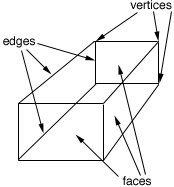SEARCH HOMEMath Central Quandaries & QueriesI am trying to help my son find the vertices, edge, and face of a 16 sided figure. I am not sure of the process to use to find these, could you please tell me how to do this? Thanks a lot, KimberlyHi Kimberly,

This is difficult to answer without knowing what you mean by a 16 sided figure. Is this a figure in the plane? Is it a 3-dimensional figure like a rectangular box? Are the "faces" flat as in the cardboard box or curved as in the surface of a soup can? The difficulty is the word face which means different things in different situations.

If you have a diagram of the 16 sided figure you can just count them. Vertices are points, edges are line segments that join the vertices and faces are the "flat" parts bounded by edges. So a rectangular box has 8 vertices, 10 edges and 6 faces. In the diagram I have identified some of them.I hope this helps,
PennyMath Central is supported by the University of Regina and The Pacific Institute for the Mathematical Sciences.